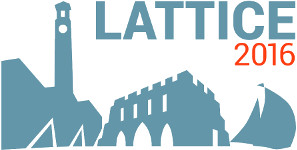#The 34th International Symposium on Lattice Field Theory (Lattice 2016)

Jul 24 – 30, 2016
Highfield Campus, University of Southampton
Europe/London timezone

## Coupled-channel analysis of $D\pi$, $D\eta$ and $D_{s}\bar{K}$ scattering using lattice QCD

Jul 28, 2016, 3:00 PM
20m
Building B2a Room 2065 (Highfield Campus, University of Southampton)

### Speaker

Dr Graham Moir (University of Cambridge)

### Description

We present an extensive study of isospin $1/2$ coupled-channel $D\pi$, $D\eta$ and $D_{s}\bar{K}$ scattering, as well as isospin $3/2$ elastic $D\pi$ scattering, from $N_{f} = 2+1$ lattice QCD. Our use of distillation in combination with variationally optimised interpolating operators allows us to extract statistically precise two-meson spectra, which we use to constrain scattering amplitudes as a function of energy. We interpret our results in terms of poles in the scattering matrix, finding a near-threshold bound state in S-wave, a deeply bound vector state in P-wave and a narrow resonance in D-wave.

### Primary author

Dr Graham Moir (University of Cambridge)

 Slides